# Tips and Tutorials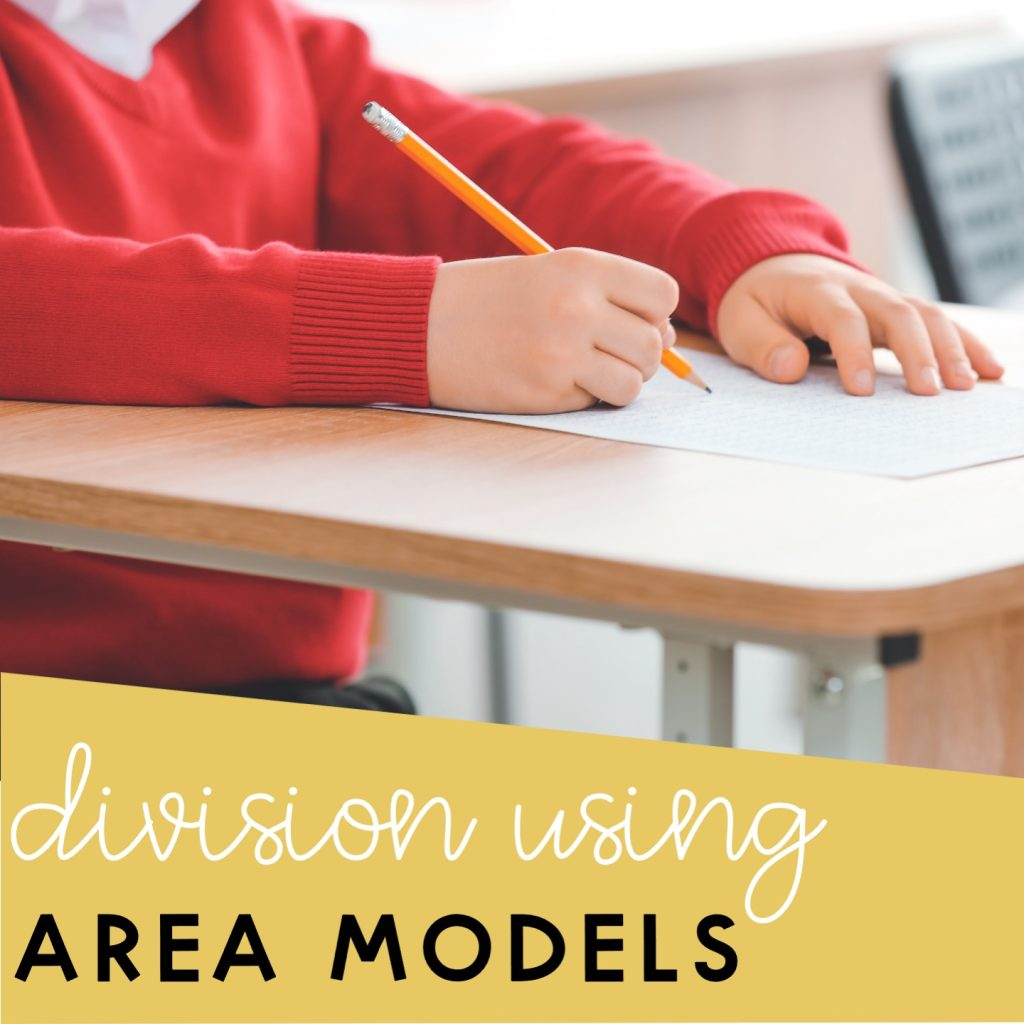## Area Model Division with and without Remainders

An area model is a powerful visual tool for a better understanding of how division works. In this blog post, you will have a guide on how to use area models to divide with remainders and without remainders. Need to know how to use area models to multiply? Check out this blog post. What are …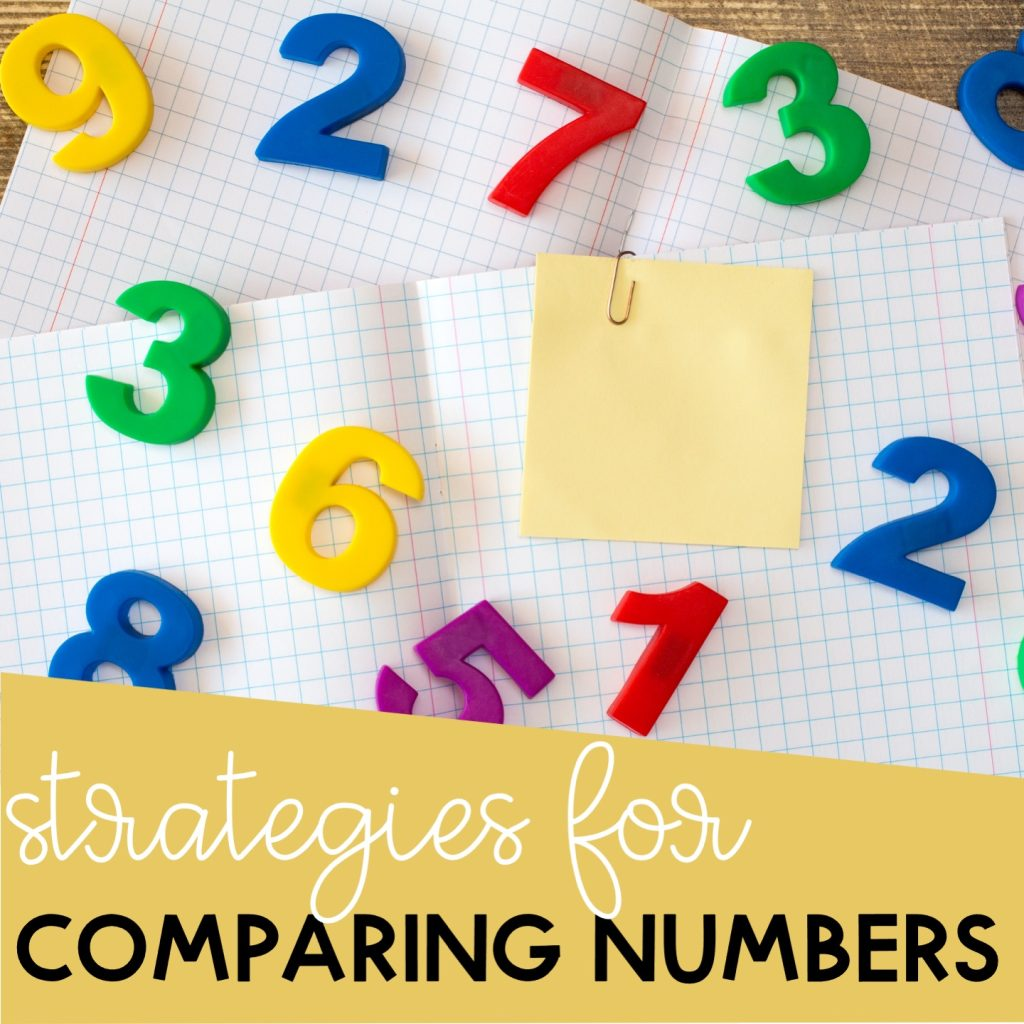## Compare Numbers Using Place Value and Number Lines

To compare numbers, you need to know the relationships between numbers. This blog post will walk you through the various strategies that will help you compare numbers. Let’s jump in! Symbols Used to Compare Numbers First, we have to understand that there are three symbols that we use when comparing numbers. We have greater than, …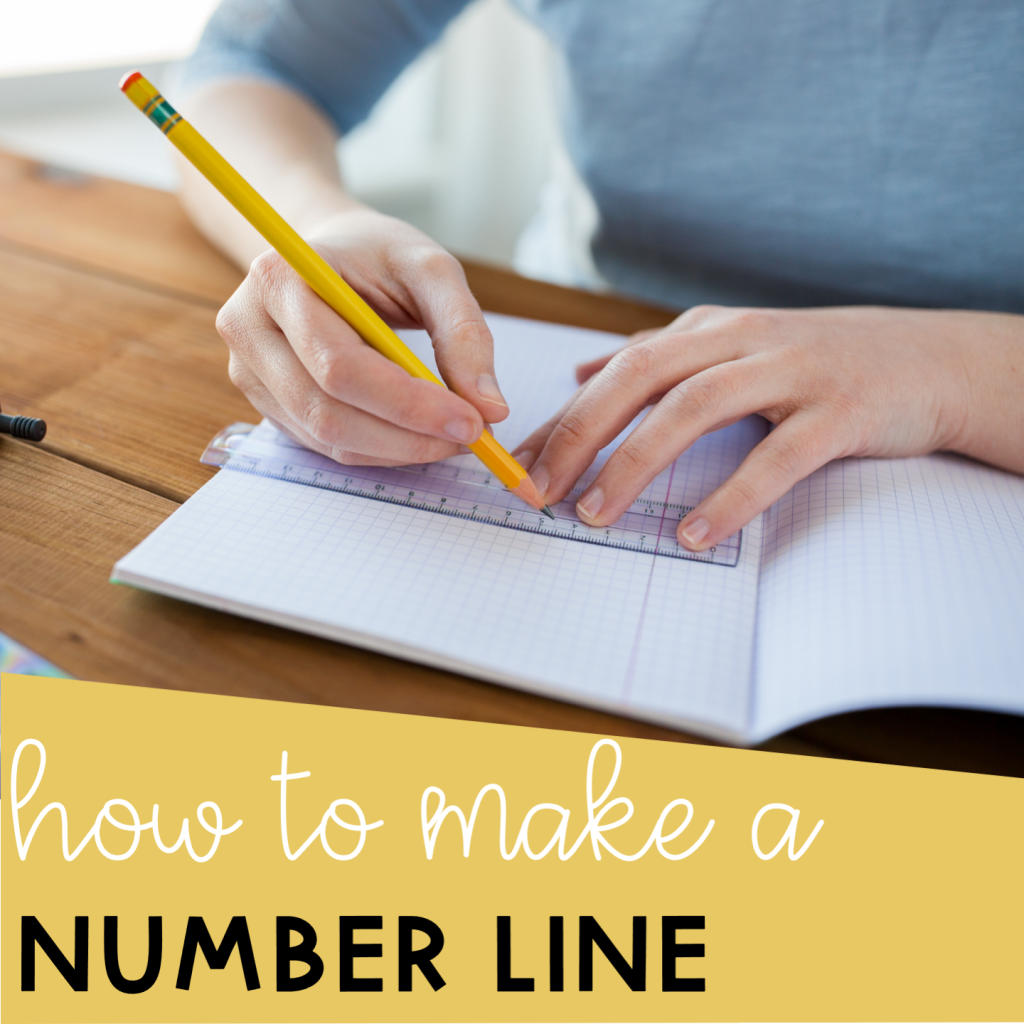## How to Make a Number Line Using PowerPoint

Do you want to know how to make a number line using PowerPoint? I will tell you how. But first, why do we need number lines? Number lines are powerful tools for students to visualize a topic. It can be used in any operation, whether addition, subtraction, multiplication, or division. Making them may seem a …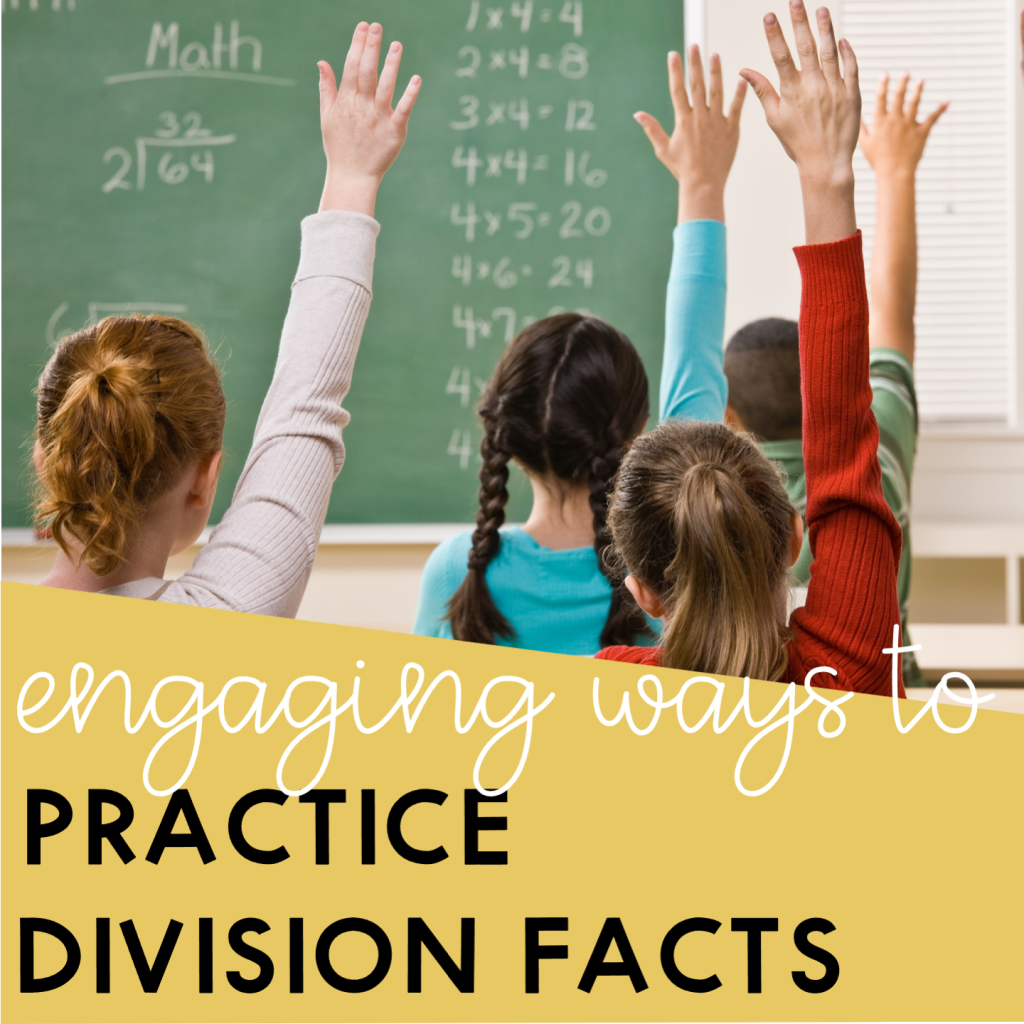## Engaging Ways to Practice Division Facts

Division facts may seem daunting for many students. It is one of the many things in Math that they will need to master in order for them to thrive. But don’t worry, learning division facts can be enjoyable. There are alternative ways to teach and practice division facts while still keeping your students engaged and …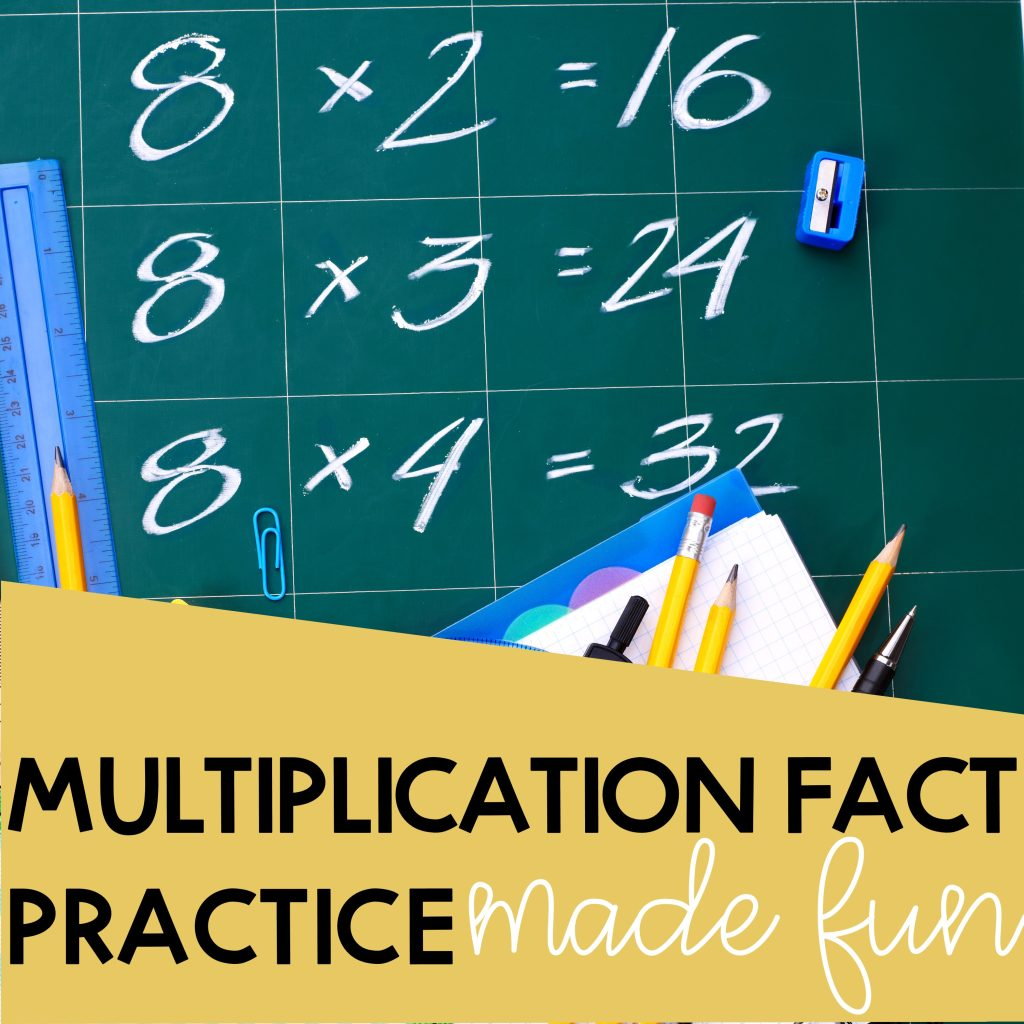## Multiplication Facts Practice Made Fun

If you need multiplication fact practice, flashcards and timed tests are not your only option. Multiplication facts are really important in elementary math. Knowing multiplication facts by heart will give elementary students the confidence to tackle more complex problems in the future. Not only that, but they are more likely to use these facts in …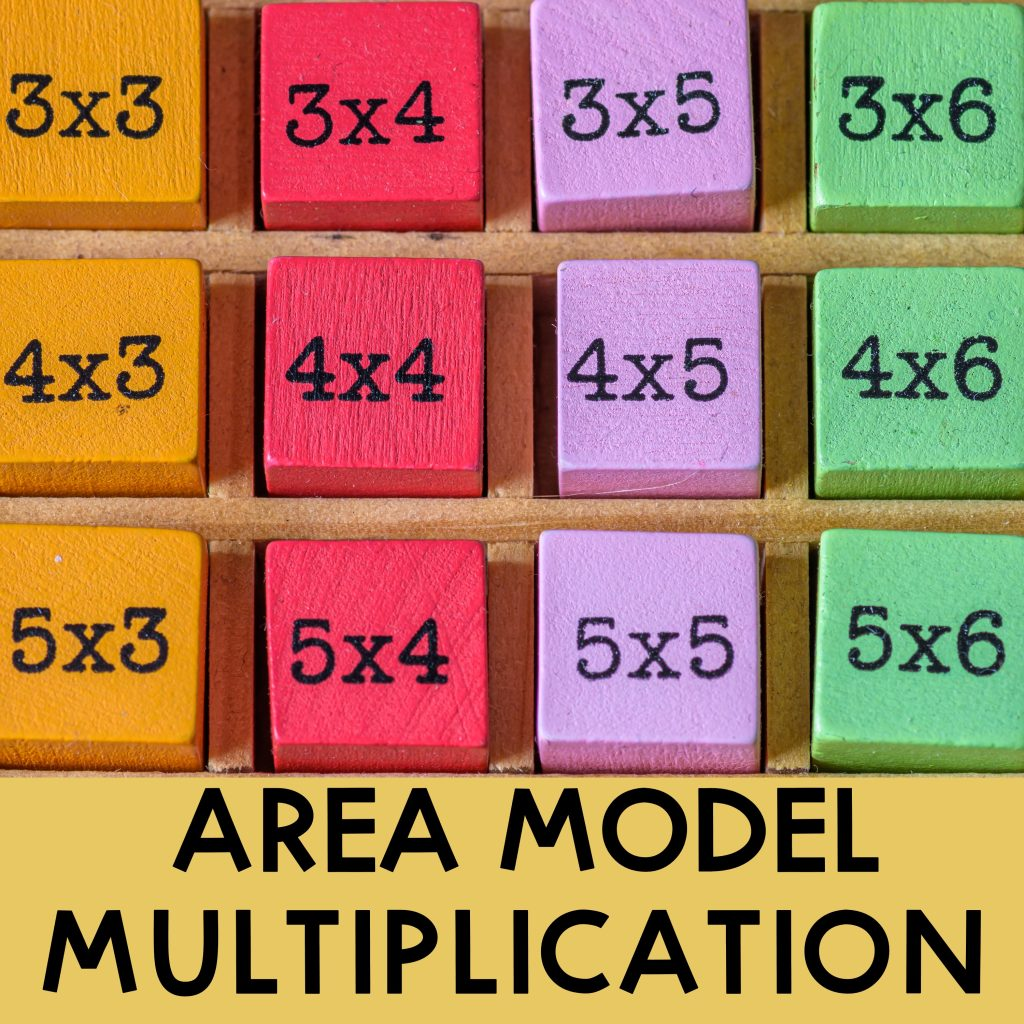## Area Model Multiplication: Guide and Examples

Area model multiplication is a great strategy when learning multi-digit multiplication. They help break down the numbers into easy-to-multiply digits and make multiplying friendly. In this article, you will learn how to use an area model to multiply 2-, 3-, and 4-digit numbers by 1-digit numbers and 2-digit numbers by 2-digit numbers. Area Model Multiplication: …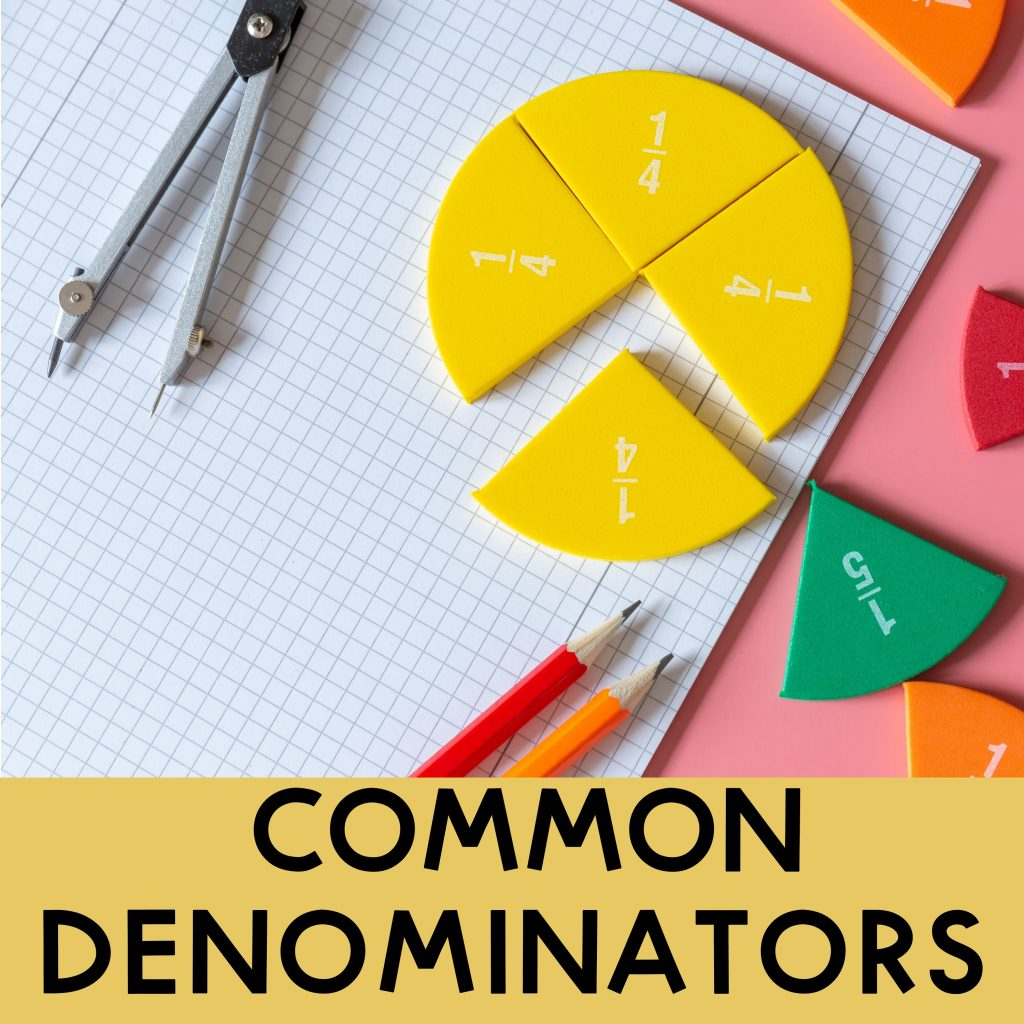## How to find common denominators in fractions?

Are you finding it difficult to find common denominators in fractions? Writing fractions with common denominators are necessary when comparing, adding, and subtracting fractions. There are two ways to find common denominators. One is by using least common multiples and another one is by using multiplication.  How do you find common denominators using least common …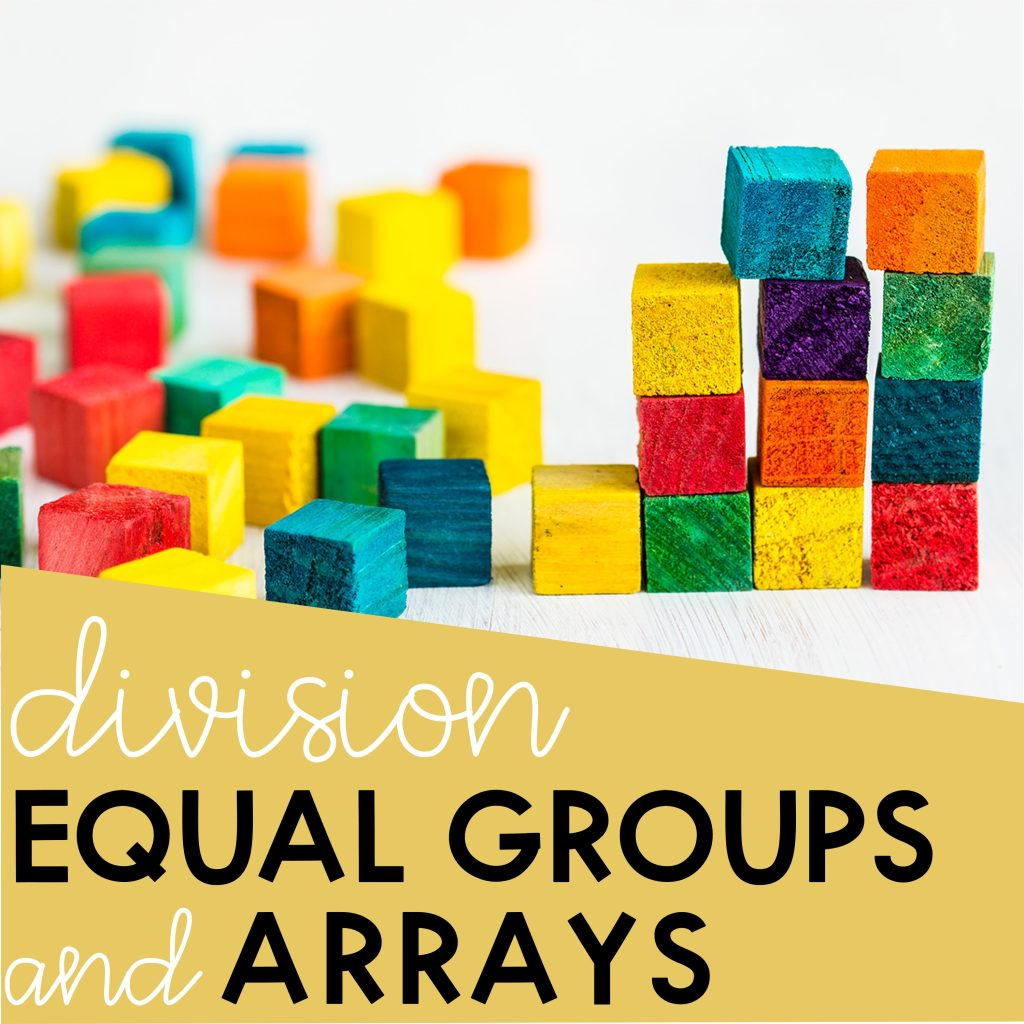## Arrays and Equal Groups in Division

Do your students need help understanding division? Arrays and equal groups are two visual models that can be used to help students understand division. Arrays An array is a visual representation of a multiplication problem but it can also be used to show a division problem. Just look at the example below.   Jackson has 12 …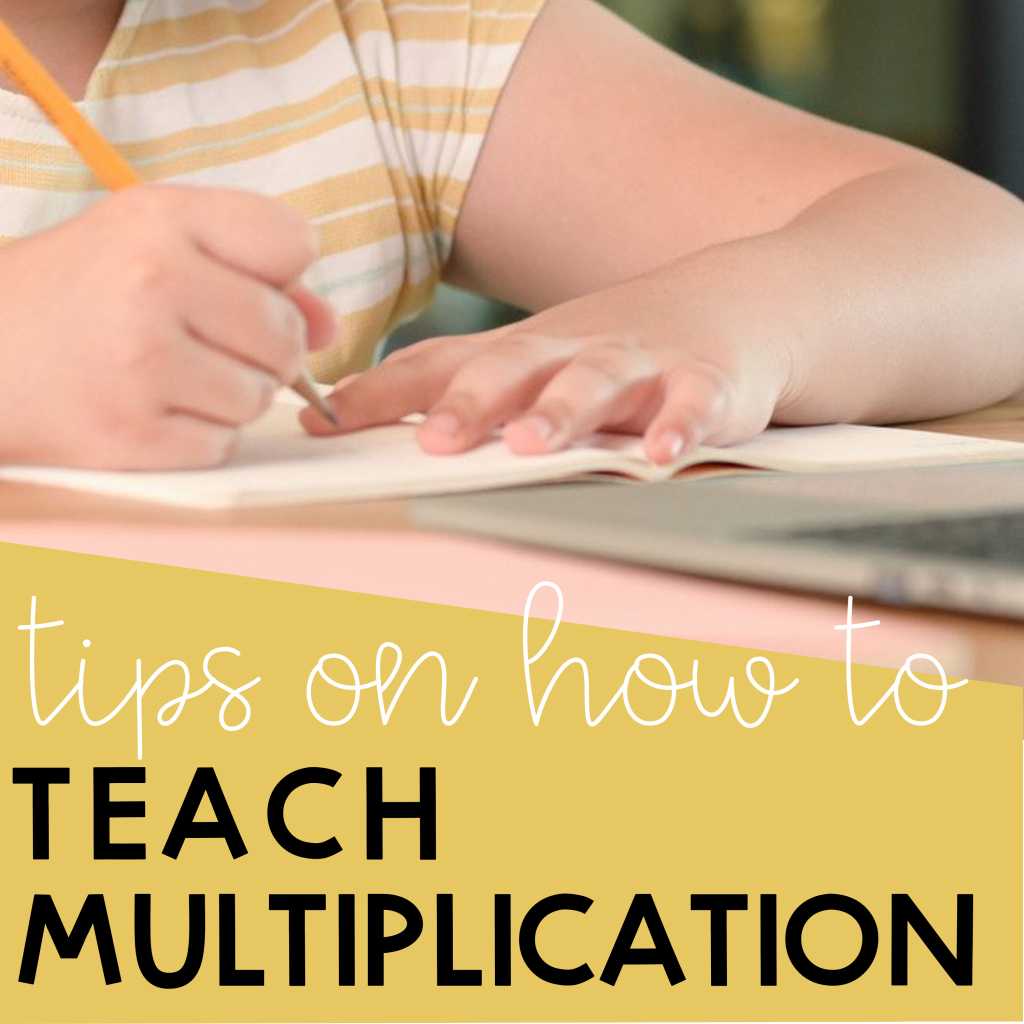## Teach Multiplication Effectively

Are your students struggling to learn their multiplication facts? Perhaps you need to take a step back and put down the multiplication table. Children learn better when they are engaged. Below are some tips you can use to teach multiplication effectively.  Tip #1: Make multiplication meaningful Oftentimes you see students struggling to learn their multiplication …